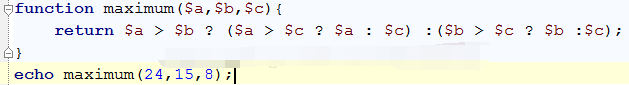• 导航
• .

# PHP判断三个数中最小 三元运算符及比较3个数的大小

PHP中可以使用三元运算符来 比较3个数的大小，这是目前能找到的用最少的代码判断三个数的大小的方法了。以下案例计算三个数中最大值。如果把大于号改成小于号就可以判断最小值

<?php

\$a = 1;
\$b = 2;
\$c = 3;
\$d = \$a > \$b ?(\$a> \$c ? \$a : \$c) :(\$b > \$c? \$b : \$c);
echo \$d;

?>```//利用三元表达式，求三个数哪个最小
function mini(\$a,\$b,\$c){
\$d=(\$a <= \$b)?(\$a <= \$c? \$a : \$c ):(\$b <= \$c? \$b : \$c );
return \$d;}
echo mini(10,20,5);```

• ^